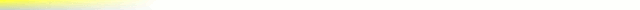# [mkgmap-dev] HGT - getElevation()

From Andrzej Popowski popej at poczta.onet.pl on Tue Jan 9 14:45:14 GMT 2018

```Hi Gerd,

I'm trying to find if resolution can be better. I think current
interpolation is not good. Imagine two combination of 4-points heights
from HGT with values like:

1 0
0 7

and

0 1
7 0

For a point in the middle of rectangle, current interpolation would give
result 0 in first case and 4 in second, while I would expect 2 in both
cases.

I think the code, which calculates interpolation based on 3 points,
should be used only if the forth is void. There could be 3 combinations
of triangles in this case. But if all 4 points are valid, there should
be applied standard bilinear interpolation. Something like:

return triangle(...
}
return triangle(...
}
return triangle(...
}
return triangle(...
}

// 4 points valid
return bilinear(...

--
Best regards,
Andrzej

```